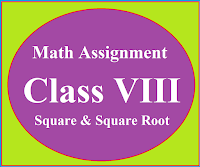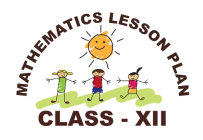### Math Assignment Class VIII | Square & Square RootMath Assignment  Class VIII | Square & Square Root Download or Print free  assignment with answer key  for   Class  8 Squares and  Square Roots.   Important and extra questions that cover all topics of square and square root and is useful and helpful for the students. Math Assignment  Class VIII | Square & Square Root LEVEL -1

### Lesson Plan Math Class 12 (Ch-5) Continuity & Differentiability

E- LESSON PLAN   SUBJECT MATHEMATICS    CLASS 10+2
Lesson Plan Class 12th Subject Mathematics for Mathematics Teacher. Effective way of Teaching Mathematics. Top planning by the teacher for effective teaching in the class.Board – CBSE CLASS –XII SUBJECT- MATHEMATICS CHAPTER 5  :-  Continuity and Differentiability

TOPIC:-
Chapter : 5 : Continuity and Differentiability

DURATION:-
This chapter is divided into ten modules and is completed in 20 class meetings.

PRE- REQUISITE KNOWLEDGE:-
Knowledge of Trigonometry class 10+1,
Knowledge Inverse Trigonometric Functions class 10+2,
Knowledge of Limits and continuity class 10+1

TEACHING AIDS:-
Green Board, Chalk,  Duster, Charts, smart board, projector, laptop etc.

METHODOLOGY:-   Lecture method

OBJECTIVES:-
• Continuity and differentiability.
• Derivative of composition functions and chain rule.
• Derivatives of inverse trigonometric functions and implicit functions.
• Concept of exponential and logarithmic functions.
• Derivative of exponential and logarithmic functions.
• Derivative of the functions expressed in parametric forms.
• Second order derivatives.
• Roll’s and mean value theorem (with proof) and their geometric interpretation.
PROCEDURE :-

Start the session by asking the questions related to the trigonometry, inverse trigonometry and limits and continuity. Now introduce the topic continuity and differentiability step by step as follows.
 S.N Topic 1 Introduction of limits and continuity, left hand limit and right hand limit with some practical problems. 2 Introduction of differentiability, left hand and right hand differentiability, with some practical problems. 3 Basic Formulas of  differentiation, differentiation of composite functions and chain rule. 4 Differentiation of inverse trigonometric functions. 5 Differentiation of implicit functions. 6 Concept of exponential and logarithmic functions. 7 Derivatives of exponential and logarithmic functions. 8 Derivatives of the functions expressed in parametric forms. 9 Concept of second order derivatives. 10 Roll’s theorem and Lagrange’s Mean Value Theorem(with proof) and their geometric interpretation.

EXPECTED OUTCOMES:-

After studying this lesson students should know the
• concept of limits, continuity and differentiability.
• Students should learn all formulas of differentiations and should know the applicability of them in different problems.
• Students should be able to find the derivatives of composite functions, inverse trigonometric functions, implicit functions, exponential functions and logarithmic functions.
• Students should know the second order derivatives and the applicability of Roll’s and mean value theorem.
STUDENTS DELIVERABLES:-
Review questions given by the teacher.
• Students should prepare the presentation on the formulas of derivatives.
• Solve NCERT problems with examples,
• Solve assignment given by the teacher.
EXTENDED LEARNING:-
Students can extend their learning in Mathematics through the RESOURCE CENTRE.  Students can also find many interesting topics on mathematics at cbsemathematics.com

ASSESSMENT TECHNIQUES:-
• Assignment sheet will be given as home work at the end of the topic.
• Separate sheets which will include questions of logical thinking and Higher order thinking skills will be given to the above average students.
• Class Test , Oral Test , worksheet and  can be made the part of assessment.
• Re-test(s) will be conducted on the basis of the performance of the students in the test.

Lesson Plan For CBSE Class 12 (Chapter 2) For Mathematics Teacher

🙏

1.